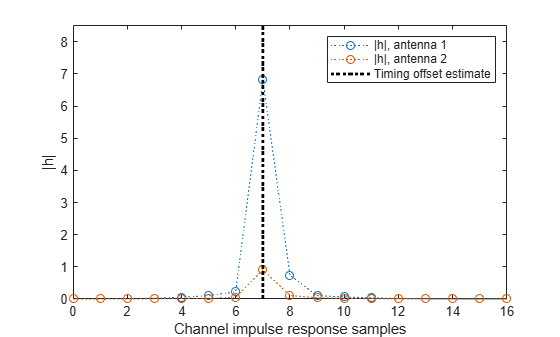# Reconstruct Channel Impulse Response Using CDL Channel Path Filters

Reconstruct the channel impulse response and perform timing offset estimation using path filters of a Clustered Delay Line (CDL) channel model with delay profile CDL-D from TR 38.901 Section 7.7.1.

Define the channel configuration structure using an `nrCDLChannel` System object. Use delay profile CDL-D, a delay spread of 10 ns, and UT velocity of 15 km/h:

```v = 15.0; % UT velocity in km/h fc = 4e9; % carrier frequency in Hz c = physconst('lightspeed'); % speed of light in m/s fd = (v*1000/3600)/c*fc; % UT max Doppler frequency in Hz cdl = nrCDLChannel; cdl.DelayProfile = 'CDL-D'; cdl.DelaySpread = 10e-9; cdl.CarrierFrequency = fc; cdl.MaximumDopplerShift = fd;```

Configure the transmit array as [M N P Mg Ng] = [2 2 2 1 1], representing 1 panel (Mg=1, Ng=1) with a 2-by-2 antenna array (M=2, N=2) and P=2 polarization angles. Configure the receive antenna array as [M N P Mg Ng] = [1 1 2 1 1], representing a single pair of cross-polarized co-located antennas.

```cdl.TransmitAntennaArray.Size = [2 2 2 1 1]; cdl.ReceiveAntennaArray.Size = [1 1 2 1 1];```

Create a random waveform of 1 subframe duration with 8 antennas.

```SR = 15.36e6; T = SR * 1e-3; cdl.SampleRate = SR; cdlinfo = info(cdl); Nt = cdlinfo.NumTransmitAntennas; txWaveform = complex(randn(T,Nt),randn(T,Nt));```

Transmit the input waveform through the channel.

`[rxWaveform,pathGains] = cdl(txWaveform);`

Obtain the path filters used in channel filtering.

`pathFilters = getPathFilters(cdl);`

Perform timing offset estimation using `nrPerfectTimingEstimate`.

`[offset,mag] = nrPerfectTimingEstimate(pathGains,pathFilters);`

Plot the magnitude of the channel impulse response.

```[Nh,Nr] = size(mag); plot(0:(Nh-1),mag,'o:'); hold on; plot([offset offset],[0 max(mag(:))*1.25],'k:','LineWidth',2); axis([0 Nh-1 0 max(mag(:))*1.25]); legends = "|h|, antenna " + num2cell(1:Nr); legend([legends "Timing offset estimate"]); ylabel('|h|'); xlabel('Channel impulse response samples');```